# Bohr Model of an Atom Electron Charge Neutral

• Slides: 35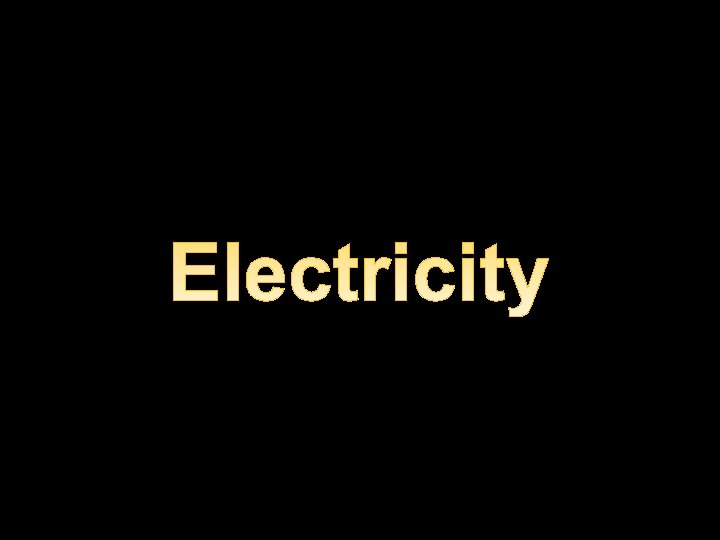Bohr Model of an Atom Electron Charge = “Neutral” Mass = 1. 674 x 10 -27 kg - Neutron - Charge = “Positive” Mass = 1. 672 x 10 -27 kg - Proton - + - - Charge = “Negative” Mass = 9. 109 x 10 -31 kg + ++ + + ++ONE COULOMB of charge (+ or -) Equals 6. 24 x 18 10 charges 6, 240, 000, 000 charges The smallest unit of charge is the charge of one electron or proton. e = 1. 60 x -19 10 CoulombsFORCE is inversely proportional to the square of the distance. F α 1 2 R FORCE is directly proportional to the product of the charges. F α q 1· q 2FORCE is inversely proportional to the square of the distance. Coulomb’s Law F α 1 2 R q · q 1 2 F α 2 R FORCE is directly proportional to the product of the charges. q 1· q 2 F =k 2 F Rα q 1· q 2 k = 8. 9875 x 109 N·m 2 / C 2 k ~ 9 x 109 N·m 2 / C 2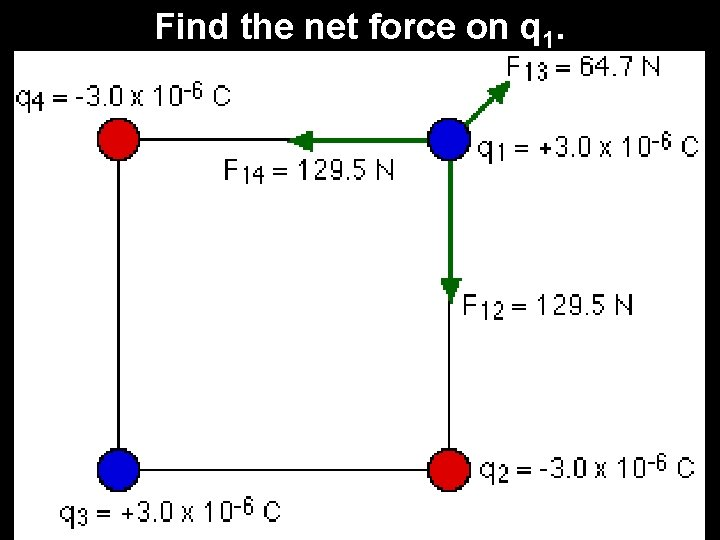Find the net force on q 1.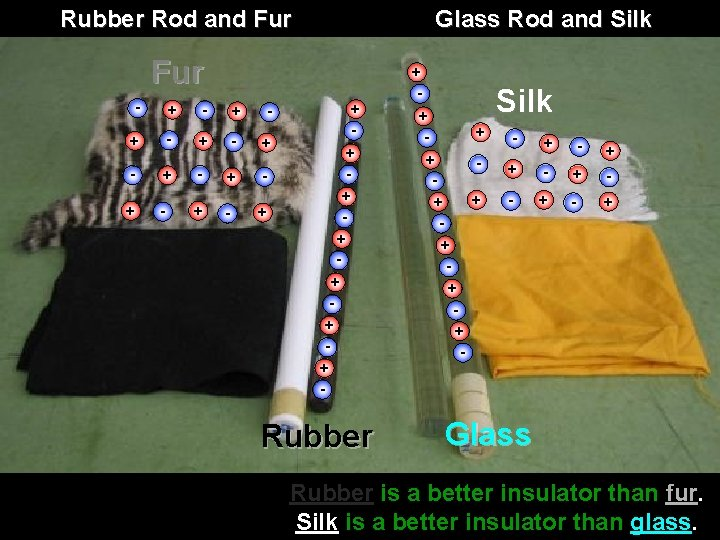Rubber Rod and Fur Glass Rod and Silk Fur - + - + - + + + + + - Rubber + + + + + - Silk - + + - - + Glass Rubber is a better insulator than fur. Silk is a better insulator than glass.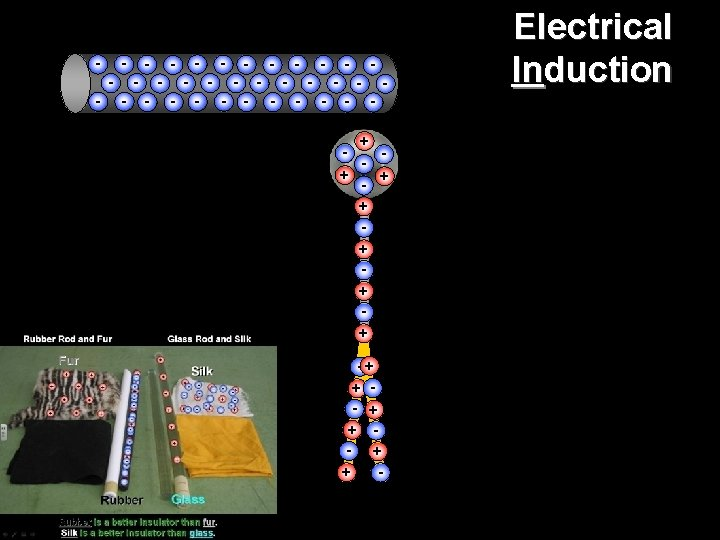- - - - + + + -+ + - + + Electrical Induction - - - - -- - - - + + + -+ + - + + Electrical Induction - - - - --+ + - + + + Electrical Induction + + + + + + + + +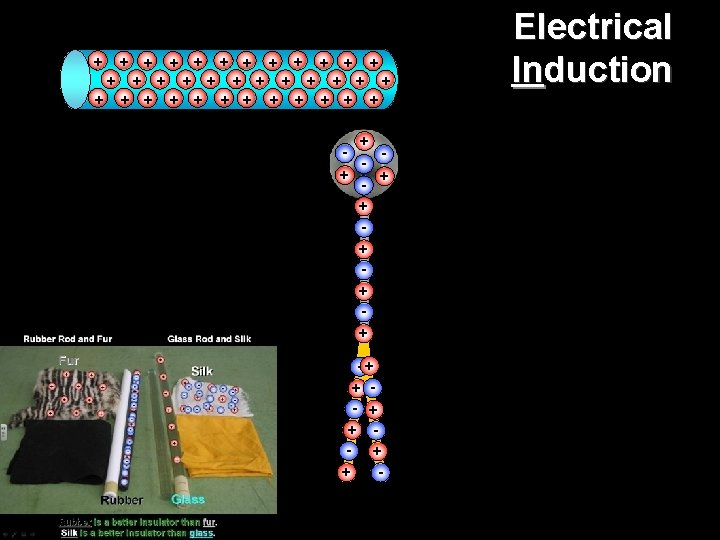-+ + - + + + Electrical Induction + + + + + + + + +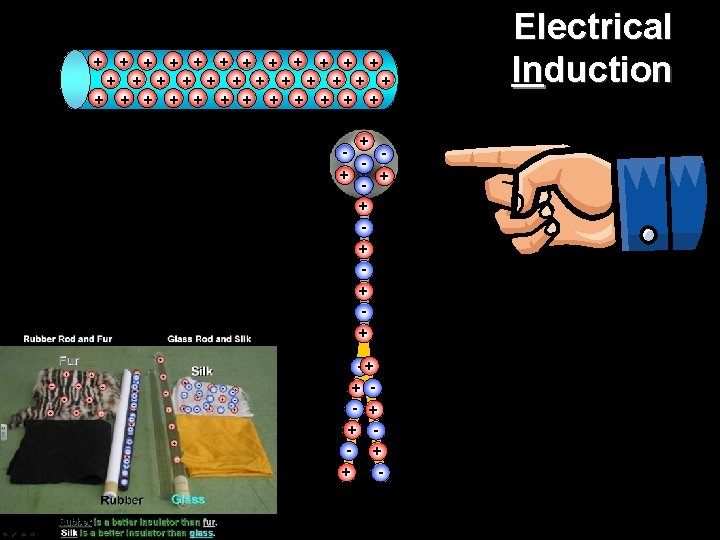-+ + - + + + Electrical Induction + + + + + + + + +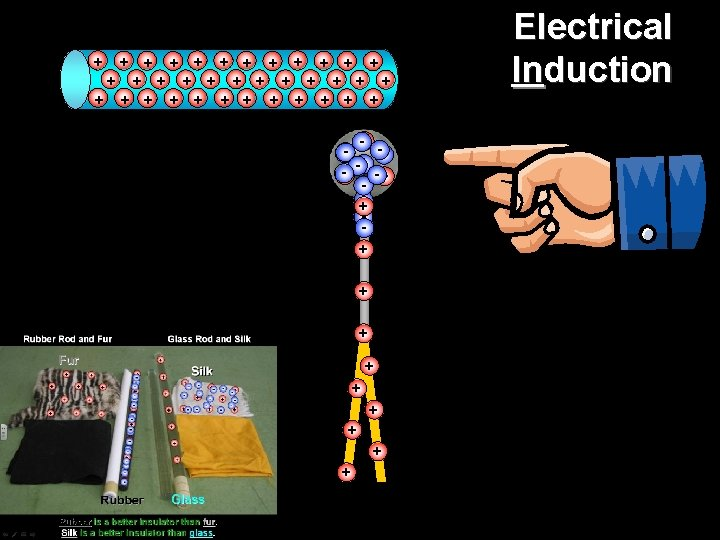-+ + - + + + Electrical Conduction + + + + + + + + +Electrical Conduction + + + +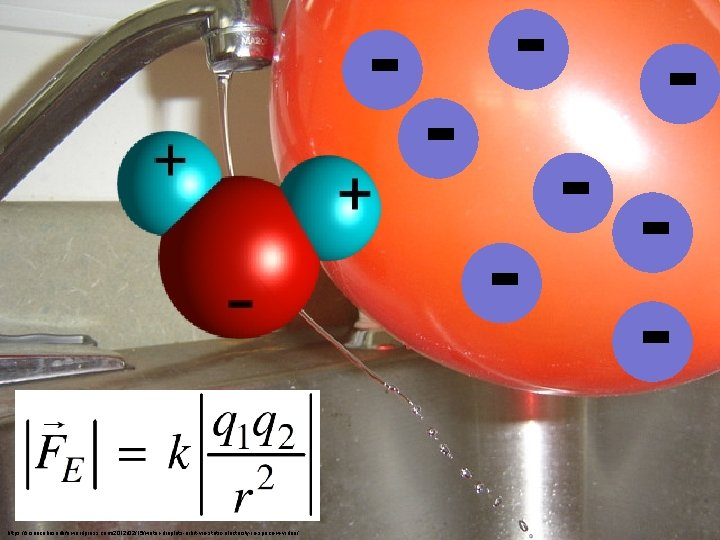- - https: //sciencebasedlife. wordpress. com/2012/02/15/water-droplets-orbit-via-static-electricity-in-space-w-video/Distance = 1 R 2 R FORCE = 1 N 1/4 N 3 R 4 R 1/9 N 1/16 N - - --- - -- -- - - - - --- - -- - -+ + GRAVITY ELECTROSTATIC FORCE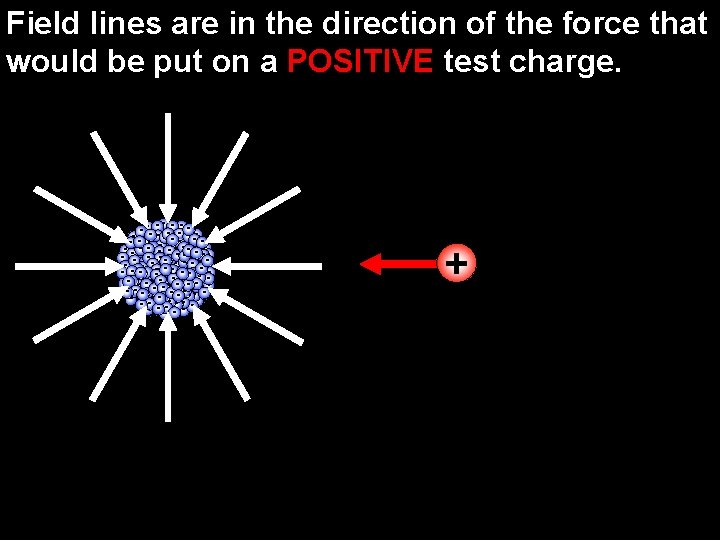+ - - -- - -- -- -- - ------- ----- - ---- -- --- --- - -- -- - -- - Field lines are in the direction of the force that would be put on a POSITIVE test charge.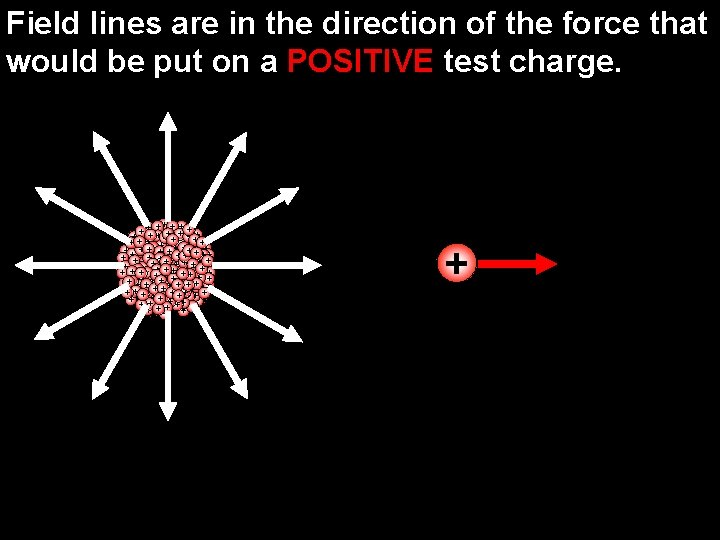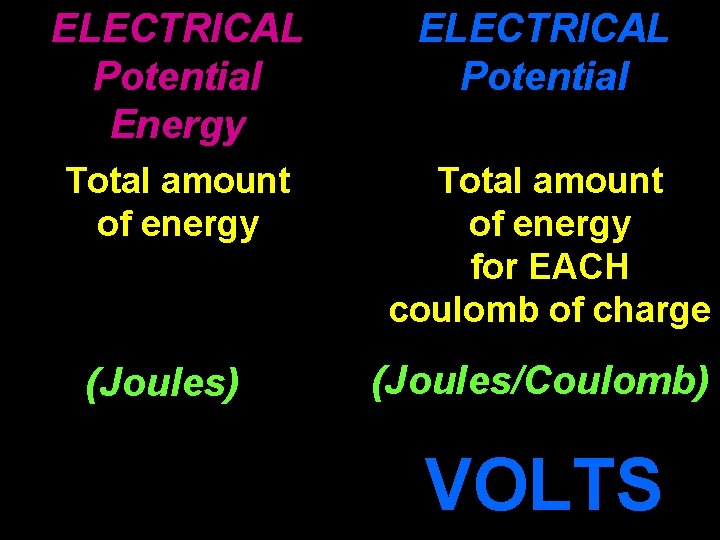ELECTRICAL Potential Energy ELECTRICAL Potential Total amount of energy for EACH coulomb of charge (Joules) (Joules/Coulomb) VOLTS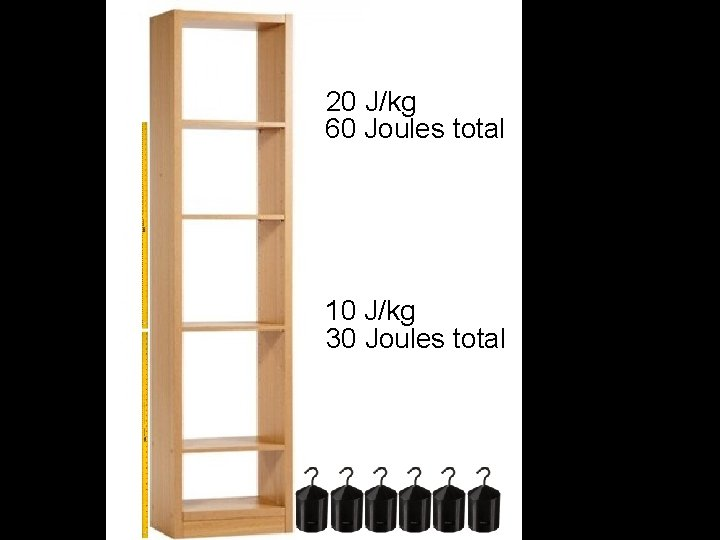20 J/kg 60 Joules total 10 J/kg 30 Joules total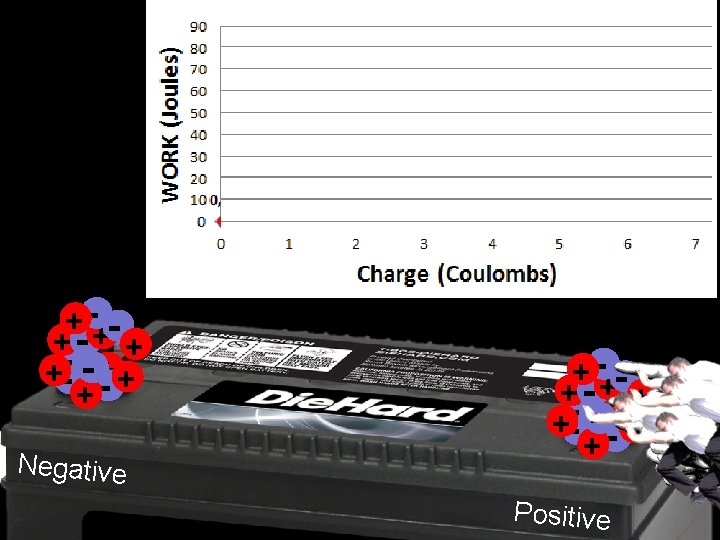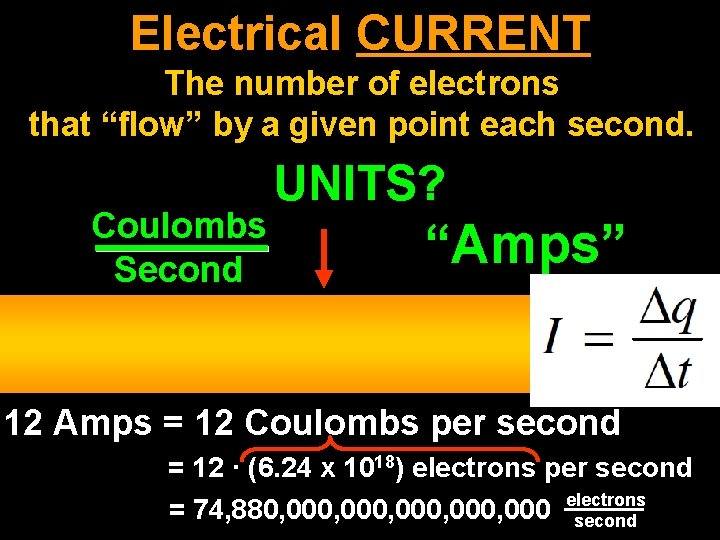Electrical CURRENT The number of electrons that “flow” by a given point each second. Coulombs Second UNITS? “Amps” 12 Amps = 12 Coulombs per second = 12 · (6. 24 x 1018) electrons per second = 74, 880, 000, 000 electrons second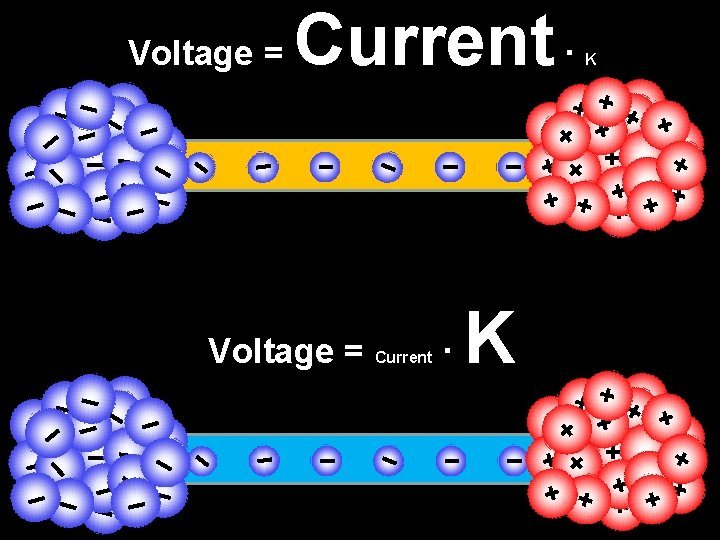Voltage = Current · K + + + ++ + + + + ++ + +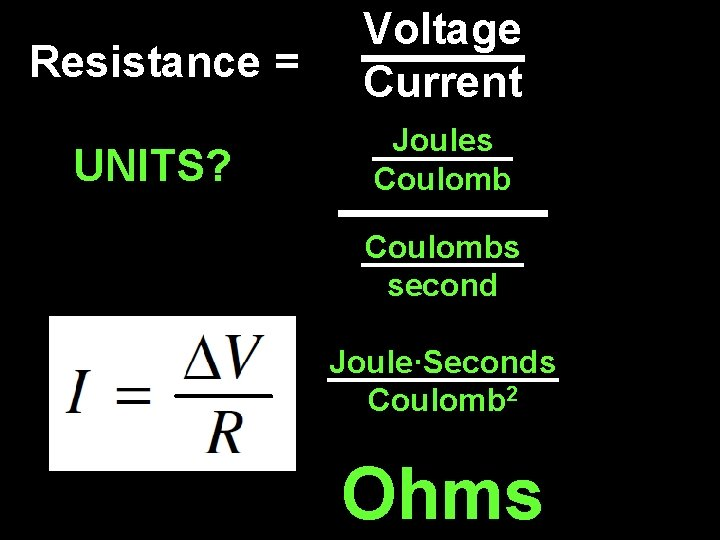Resistance = UNITS? Voltage Current Joules Coulombs second Joule·Seconds Coulomb 2 Ohms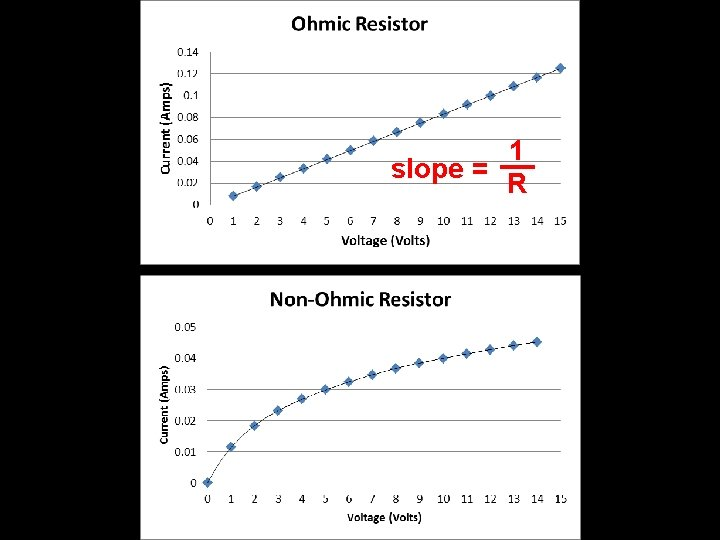1 slope = R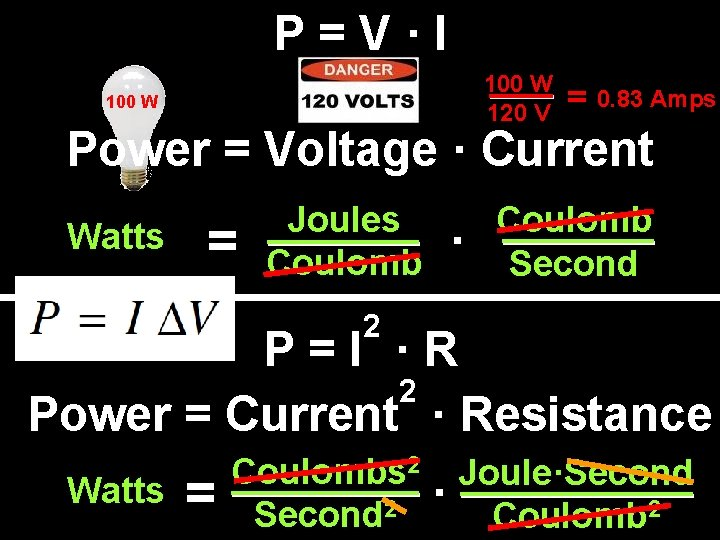P=V·I 100 W 120 V 100 W = 0. 83 ? Amps Power = Voltage · Current Watts = Joules Coulomb · Coulomb Second 2 P=I ·R 2 Power = Current · Resistance Watts = Coulombs 2 Second 2 Joule·Second · Coulomb 2"Resistivity geometry" by User: Omegatron - Created by User: Omegatron using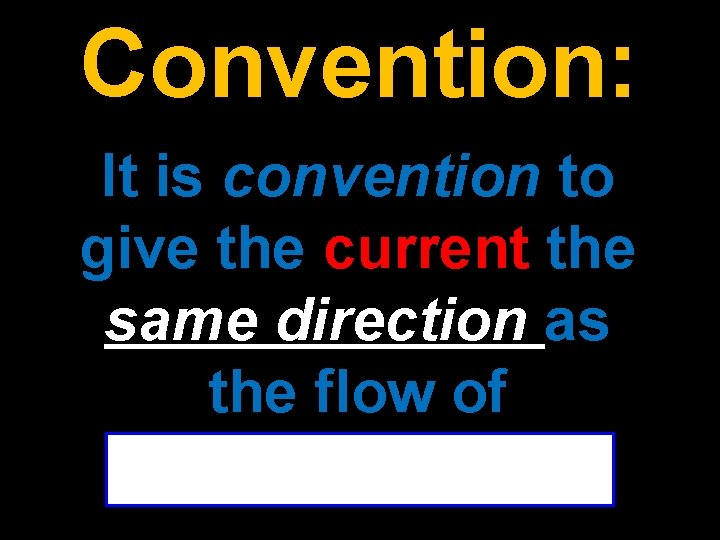Convention: It is convention to give the current the same direction as the flow of positive charge!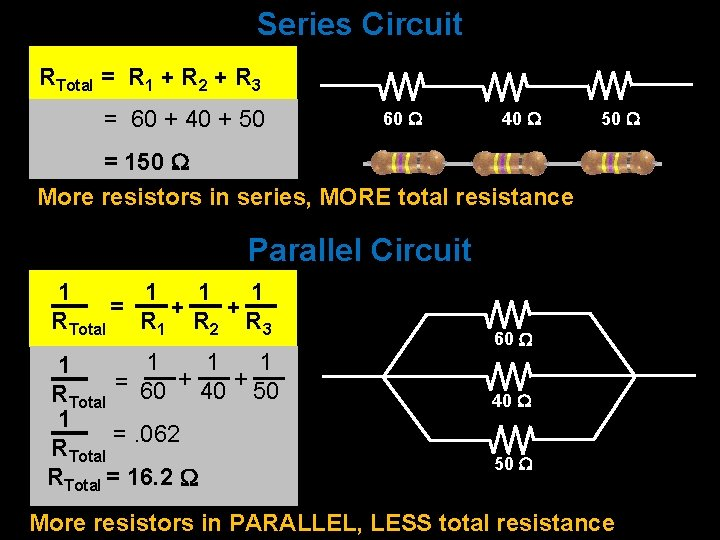Series Circuit RTotal = R 1 + R 2 + R 3 = 60 + 40 + 50 60 40 50 = 150 More resistors in series, MORE total resistance Parallel Circuit 1 1 = + + RTotal R 1 R 2 R 3 1 1 = 60 + 40 + 50 RTotal 1 =. 062 RTotal = 16. 2 60 40 50 More resistors in PARALLEL, LESS total resistance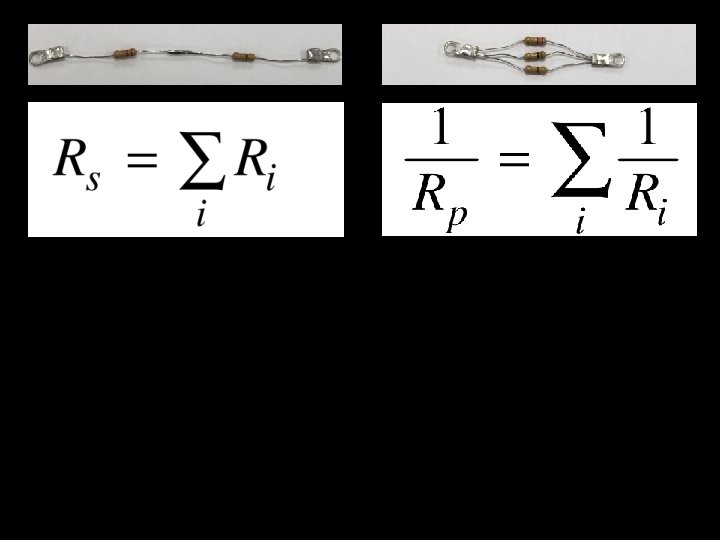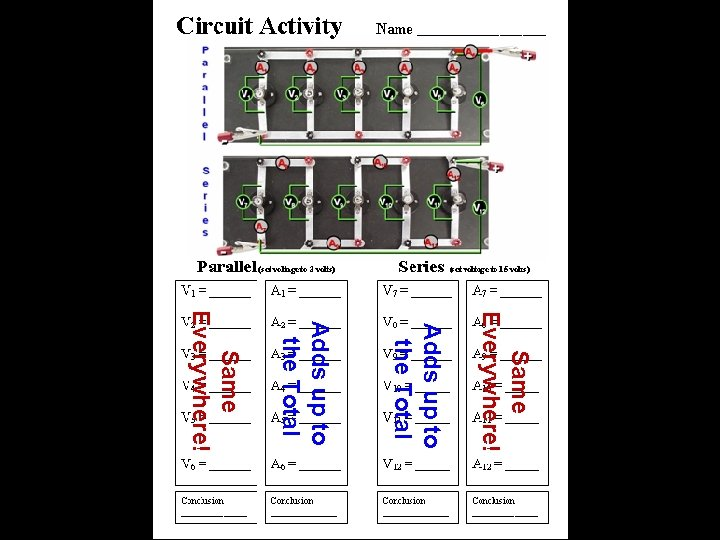Same Everywhere! Adds up to the Total Same Everywhere!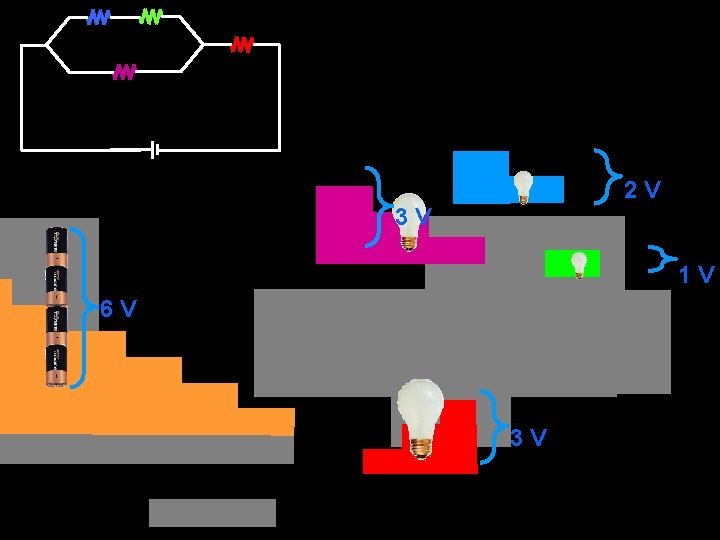2 V 3 V 1 V 6 V 3 V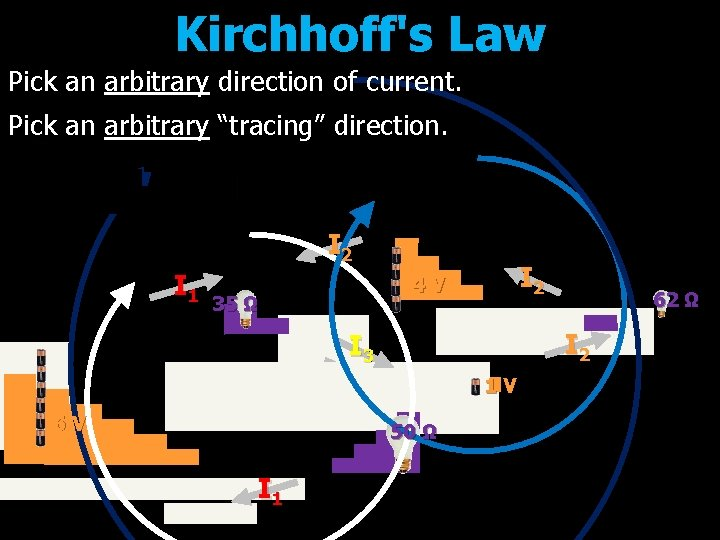Kirchhoff's Law Pick an arbitrary direction of current. Pick an arbitrary “tracing” direction. I 2 I 1 I 2 4 V 35 Ω I 2 I 3 1 V 6 V 50 Ω I 1 62 Ω6. 0 Volts Internal Resistance 5. 0 Volts